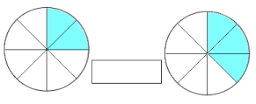2 and 1/8th plus 1 and 1/3rd =

x =  3.4583

### Step-by-step explanation:Did you find an error or inaccuracy? Feel free to write us. Thank you!Tips to related online calculators
Need help to calculate sum, simplify or multiply fractions? Try our fraction calculator.

## Related math problems and questions:

• Fractions and mixed numerals(a) Convert the following mixed numbers to improper fractions. i. 3 5/8 ii. 7 7/6 (b) Convert the following improper fraction to a mixed number. i. 13/4 ii. 78/5 (c) Simplify these fractions to their lowest terms. i. 36/42 ii. 27/45 2. evaluate the followAdd two mixed fractions: 2 4/6 + 1 3/6
• Evaluate mixed expressionsWhich of the following is equal to 4 and 2 over 3 divided by 3 and 1 over 2? A. 4 and 2 over 3 times 3 and 2 over 1 B. 14 over 3 times 2 over 7 C. 14 over 3 times 7 over 2 D. 42 over 3 times 2 over 313 3/4 + 2 3/5 + 5 1/2 Show your solution.
• Difference mixed fractionsWhat is the difference between 4 2/3 and 3 1/6?
• Honey 3Honey jogged 1 3/4 km on Monday,1 1/2 km on Wednesday and 1  2/3 km on Friday . how far did he jog?
• Simplify 3Simplify mixed numerals expression: 8 1/4- 3 2/5 - (2 1/3 - 1/4) Show your solution.Why does 1 3/4 + 2 9/10 equal 4.65? How do you solve this?
• SubtractSubtract two mixed numbers: 3 1/2 -1 3/4
• Simplify 7Simplify. 7-1/3÷ 3-2/3 of 2+ 4-1/2÷ 2-1/4+ 1/2 solution and by step by step
• EvaluateThe division of numbers $a and$b increase by-product of the numbers $c and$d
• Mixed numbersRewrite mixed numbers, so the fractions have the same denominator: 5 1/5 - 2 2/3
• Equivalent fractions 2Write the equivalent multiplication expression. 2 1/6÷3/4
• Divide 11Divide the product of 4 and 5/8 by 1 1/2. Write your answer as a mixed number.
• Team runThe first team member in a 926-person relay race must run 2 1/4 laps, the second team member must run 1 1/2 laps, and the third team member must run 3 1/4 laps. How many laps in all must each team run?Add this two mixed numbers: 1 5/6 + 2 2/11=What is 4 1/2+2/7-213/14?